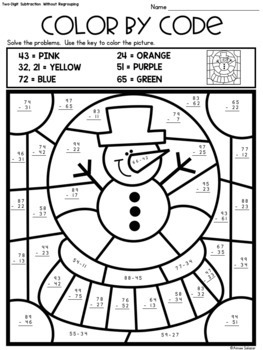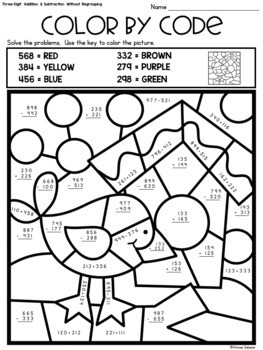# Winter Color by Code - Two & Three-Digit Addition & Subtraction

Rated 4.81 out of 5, based on 330 reviews
330 Ratings;
2nd - 3rd
Subjects
Resource Type
Standards
Formats Included
• PDF
Pages
18 pages
Report this resource to TPT

### Description

Color by code activities are an engaging way to give your students practice with their two and three-digit addition and subtraction skills. These coloring pages are more than just art. They give students skill based practice, while incorporating art.

Students will solve the problems in each section of the coloring page. Then, they will use the key (found on each page) to color in each section.

This set includes the following skills:
*Two-Digit Subtraction WITHOUT Regrouping
*Two-Digit Addition and Subtraction WITHOUT Regrouping

*Two-Digit Addition WITH and WITHOUT Regrouping
*Two-Digit Subtraction WITH and WITHOUT Regrouping
*Two-Digit Addition and Subtraction WITH and WITHOUT Regrouping

*Three-Digit Subtraction WITHOUT Regrouping
*Three-Digit Addition and Subtraction WITHOUT Regrouping

*Three-Digit Addition WITH and WITHOUT Regrouping
*Three-Digit Subtraction WITH and WITHOUT Regrouping
*Three-Digit Addition and Subtraction WITH and WITHOUT Regrouping

*Two and Three-Digit Addition and Subtraction WITH and WITHOUT Regrouping (2 different pages included)

NOTE: The images used for the two-digit addition and subtraction skills listed above are also used for the three-digit addition and subtraction skills. The images used for the two and three-digit addition and subtractions skills pages are not repeated in the set.

Be sure to check out the preview. :)

Total Pages
18 pages
N/A
Teaching Duration
N/A
Report this resource to TPT
Reported resources will be reviewed by our team. Report this resource to let us know if this resource violates TPT’s content guidelines.

### Standards

to see state-specific standards (only available in the US).
Fluently add and subtract within 100 using strategies based on place value, properties of operations, and/or the relationship between addition and subtraction.
Add up to four two-digit numbers using strategies based on place value and properties of operations.
Add and subtract within 1000, using concrete models or drawings and strategies based on place value, properties of operations, and/or the relationship between addition and subtraction; relate the strategy to a written method. Understand that in adding or subtracting three-digit numbers, one adds or subtracts hundreds and hundreds, tens and tens, ones and ones; and sometimes it is necessary to compose or decompose tens or hundreds.